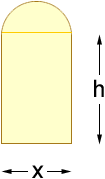Name: Erin Who is asking: Other Level: Secondary Question: A Norman window has the shape of a rectangle surmounted by a semicircle. If the perimeter of the window is 30 ft, express the atrea, A, as a function of the width x of the window. Hi Erin, Draw a diagram.Write the radius of the circle, r, in terms of x. The perimeter is x 30 ft, hence, x + 2h + (half the circumference of a circle of radius r) = 30 ft. Solve this equation for y. The area of the window is xy + half the area of a curcle of radius r. Cheers, Penny Go to Math Central